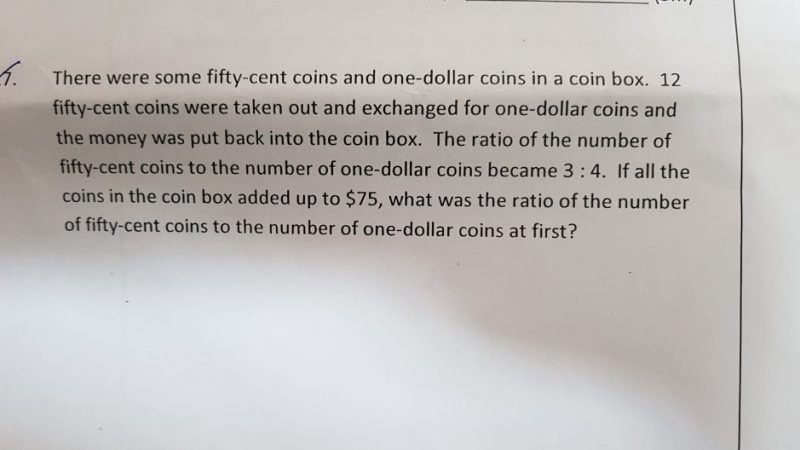QuestionHi! Can anyone help me with this qns? Thanks!

After exchange:

#50Cents : #1Dollar = 3 : 4

valueOf50Cents : valueOf1Dollars = 1.5 : 4 = 3 : 8

Therefore, out of \$75,

valueOf50Cents = \$75 ÷ (3+8) x 3 ~~~~~~~~~~~~~~

This question the ratio (3:4) is not realistic.

3:4 is 75%

If there are \$20 from 50 cents, there will be \$55 from \$1. Then the number ratio is 40:55, which is around 72.73%.

However, if there are \$21 from 50 cents, there will be \$54 from \$1. Then the number ratio is 42:54, which is around 77.78%.

What do you think? Please do reply me if I am missing anything. Many thanks.

0 Replies 0 Likes